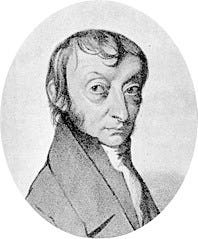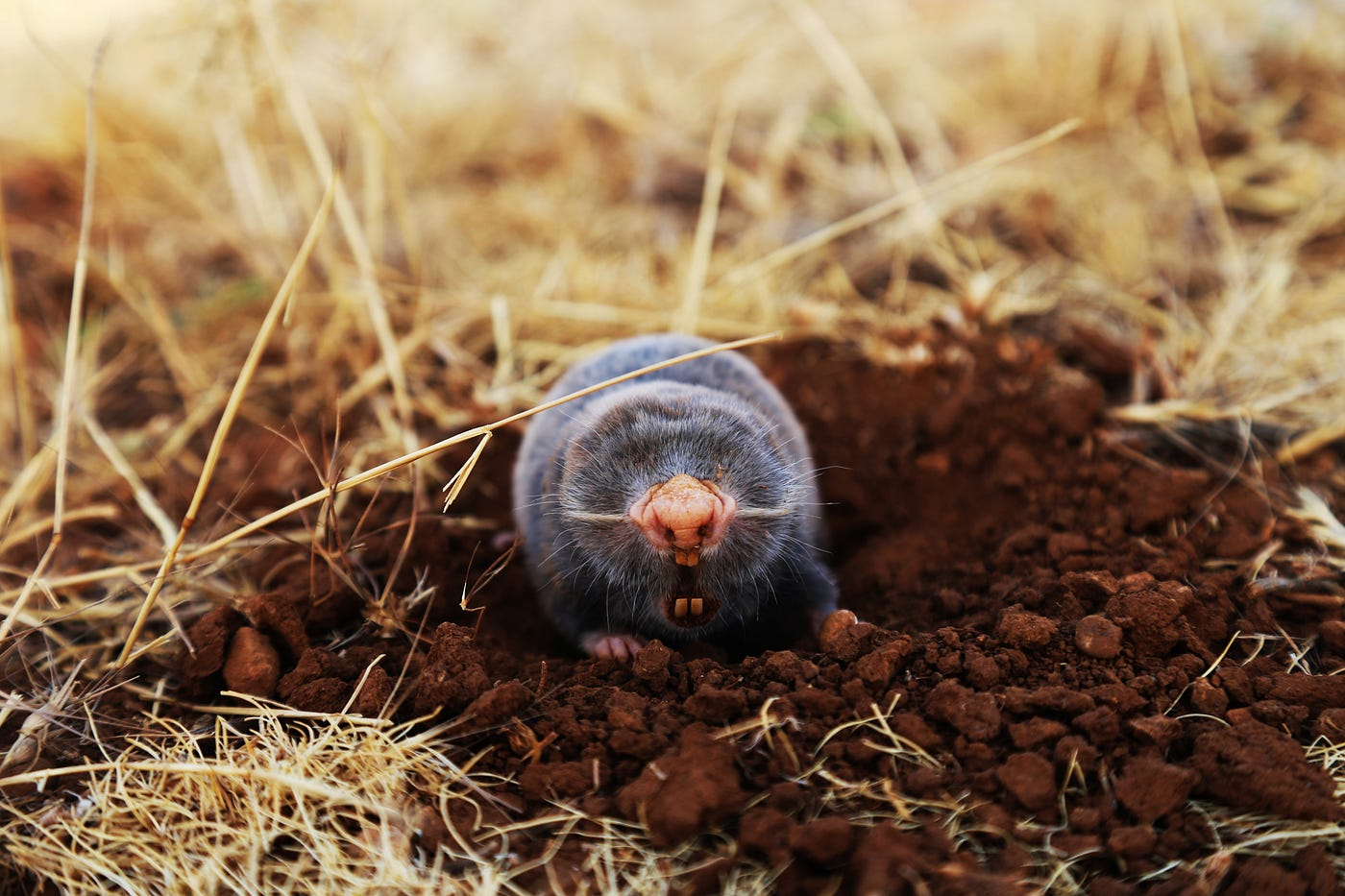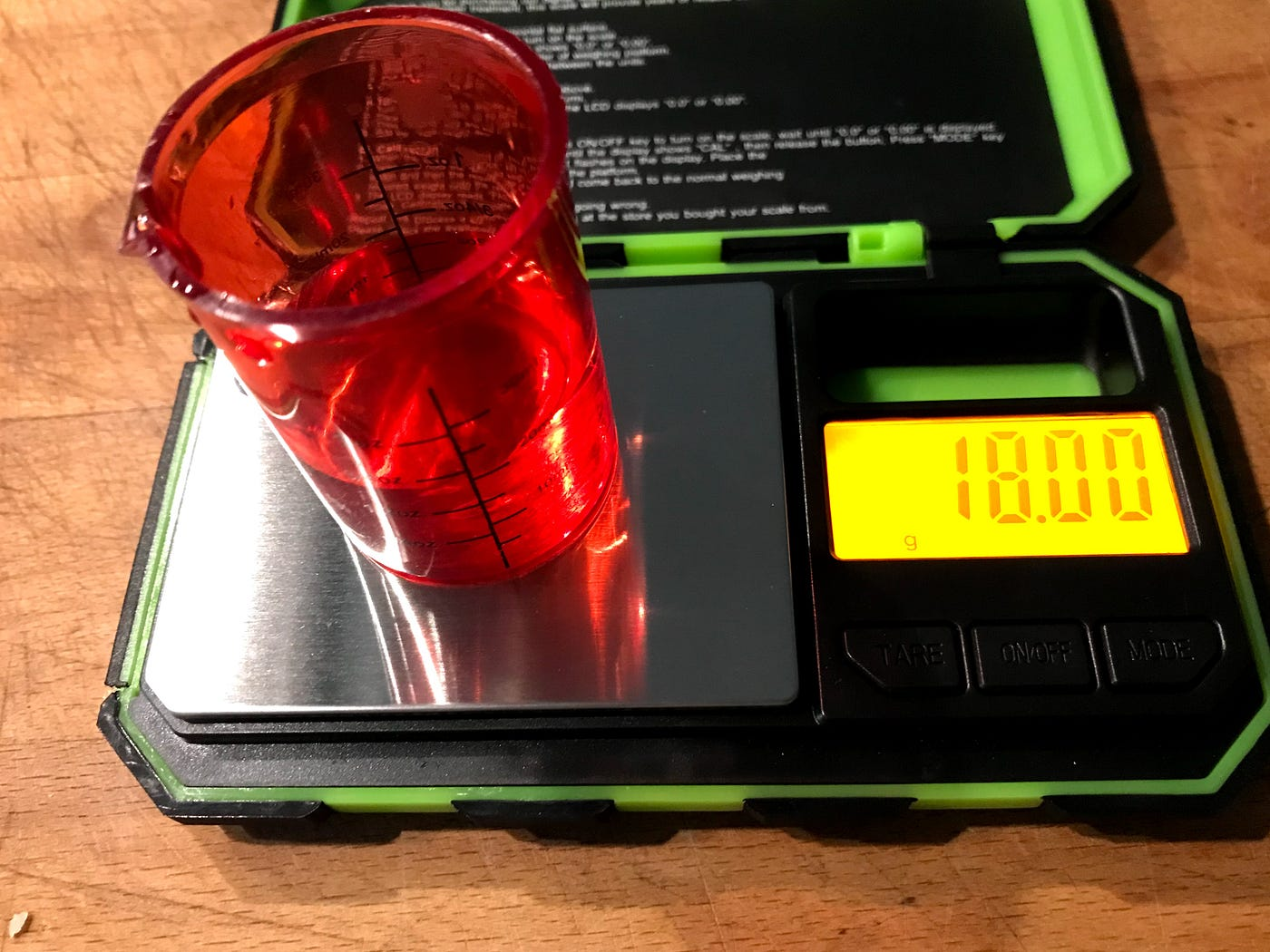# We Are All Made Of Stars

## Avogadro’s number did not come from Avogadro

In 1811, the Italian chemist and physicist Amedeo Avogadro stated his famous law:

Equal volumes of gas, in the same temperature and pressure conditions, contain the same number of molecules.

But how many molecules?

The short answer is: a lot.

The long answer is: well, quite a lot.Amedeo Avogadro | From a drawing by C. Sentier, executed in Torino at Litografia Doyen in 1856, Public domain, via Wikimedia Commons

Avogadro himself didn’t know the answer. In fact, he did not compute his eponymous number. It was calculated at the beginning of twentieth century and represents the number of things contained in a quantity called a mole.

## Not the mole you are thinking of

But what does all this actually mean?Not this kind of mole | Photo by ahmad kanbar on Unsplash

Long story short: a mole of atoms, electrons, molecules, dogs, bats… contains always the same number of atoms, electrons, molecules, dogs, bats… that is 6.022x10²³.

From the definitions of mole and atomic weight, if an atom has atomic weight of x, a mole of those atoms will weigh x grams. So, if you look at the periodic table of elements and look at the atomic weight for helium, it’s 4 and for carbon is 12. This means that a mole of helium will weight 4 g, a mole of carbon will weight 12 g, and so on (well, the exact atomic and molar weight are slightly different because you have to consider the isotopes, but let’s keep it simple).

Going from atoms to molecules we can do the same calculations, replacing the atomic weight with the molecular one.

## How many molecules are “a lot?”

We know that a mole of pure water weights 18g because molecular weight of water is 18 (water formula is H2O, so weight is 1+1+16=18), so in 18 g of water there are one number of Avogadro of molecules, that is 6.022x10²³.18g of water contain one number of Avogadro of molecules | Picture by the author.

Scientific notation is useful for make calculations but cannot give a clear estimation of how big a number is; one number of Avogadro in fact is:

602 200 000 000 000 000 000 000

Scientists estimate that the entire universe contains between 10²² to 10²⁴stars, which means:

there are more molecules in a bottle of water than stars in the whole universe!

Moreover, if you calculate the age of the universe in seconds, the result is around 4x10¹⁷, that means:

there are more molecules in a bottle of water than seconds in the life of the universe!

As last comparison, the total number of homo sapiens born on Earth since the species appearance 50 000 years ago is around 108 000 000 000, that means:

there are more molecules in a bottle of water than the total number of human beings who ever lived on Earth!

So, “a lot” is really quite a lot.

## Something to listen

--

--## Diego Tonini

Writer and science communication enthusiast, I think science can be fun and everyone can understand it!